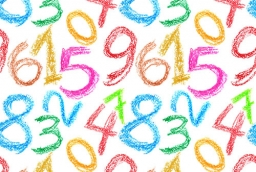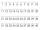# Three-digit numbers

How many are all three-digit numbers that are made up of digits 0,2,5,7 and are divisible by 9 if the digits can be repeated?

n =  7

### Step-by-step explanation:Did you find an error or inaccuracy? Feel free to write us. Thank you!Tips to related online calculators
Would you like to compute count of combinations?

## Related math problems and questions:

• Divisible by fiveHow many different three-digit numbers divisible by five can we create from the digits 2, 4, 5? The digits can be repeated in the created number.
• PermutationsHow many 4-digit numbers can be composed of numbers 1,2,3,4,5,6,7 if: and the digits must not be repeated in the number b, the number should be divisible by five, and the numbers must not be repeated c, digits can be repeated
• Phone numbersHow many 7-digit telephone numbers can be compiled from the digits 0,1,2,..,8,9 that no digit is repeated?
• Three-digit integersHow many three-digit natural numbers exist that do not contain zero and are divisible by five?
• DigitsHow many odd four-digit numbers can we create from digits: 0, 3, 5, 6, 7? (a) the figures may be repeated (b) the digits may not be repeated
• Three digits numberFrom the numbers 1, 2, 3, 4, 5 create three-digit numbers that digits not repeat and number is divisible by 2. How many numbers are there?
• Three-digit numbersWe have digits 0,1,4,7 that cannot be repeated. How many three-digit numbers can we write from them? You can help by listing all the numbers.
• NumbersHow many different 3 digit natural numbers in which no digit is repeated, can be composed from digits 0,1,2?
• DigitsHow many five-digit numbers can be written from numbers 0.3,4, 5, 7 that is divided by 10, and if digits can be repeated?
• How many 4How many four-digit numbers that are divisible by ten can be created from the numbers 3, 5, 7, 8, 9, 0 such no digits repeats?
• Five-digit numberAnna thinks of a five-digit number that is not divisible by three or four. If he increments each digit by one, it gets a five-digit number that is divisible by three. If he reduces each digit by one, he gets a five-digit number divisible by four. If it sw
• Dd 2-digit numbersFind all odd 2-digit natural numbers compiled from digits 1; 3; 4; 6; 8 if the digits are not repeated.
• Five-digitFind all five-digit numbers that can be created from numbers 12345 so that the numbers are not repeated and then numbers with repeated digits. Give the calculation.
• How manyHow many double-digit numbers greater than 30 we can create from digits 0, 1, 2, 3, 4, 5? Numbers cannot be repeated in a two-digit number.
• Inverted nineIn the hotel Inverted Nine, each hotel room number is divisible by 6. How many rooms can we count with the three-digit number registered by digits 1,8,7,4,9?
• DigitsHow many natural numbers greater than 4000 which are formed from the numbers 0,1,3,7,9 with the figures not repeated, B) How many will the number of natural numbers less than 4000 and the numbers can be repeated?
• CombinationsHow many different combinations of two-digit number divisible by 4 arises from the digits 3, 5 and 7?